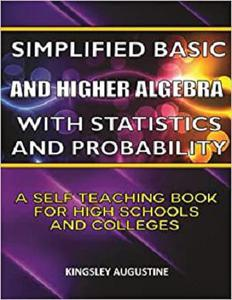Simplified Basic and Higher Algebra with Statistics and Probability: A Self Teaching Book for High Schools and CollegesEnglish | September 7, 2021 | ISBN: | ASIN: B09FNS2P14 | 471 pages | PDF | 4.12 Mb

If you have bought any of my books on Simplified Algebra (Volume 1 to 4), then this book might not be for you because this book is an edited version with some corrections and inclusion of solutions to the exercises. However, if you have not bought any of my books on Simplified Algebra (Volume 1 to 4), then you will find this book very useful for learning the basics and more advanced problems on Algebra including basic problems on statistics and probability.
Simplified Basic and Higher Algebra with Statistics and Probability: A Self-Teaching Book for High Schools and Colleges provides an easy way to gain a solid understanding of the basics and more difficult problems on algebra and problems in statistics and probability in Mathematics. Assuming no background knowledge of the subject, this clear and self teaching guide explains solved problems in ways that are easy to understand. Exercises are given at the end of each chapter for students to use to asses their understanding of the topics. Solutions to the exercises are provided at the end of the book.
This maths book is an ideal resource for high school students and college students taking algebra, statistics and probability courses, for students taking higher level courses needing to refresh their knowledge, and for those preparing for admission and need to take entrance test or exam.
Topics covered in this e-book include:
Basic arithmetic operations
Laws of indices
Change of subject of formulae
Simultaneous equations
Word problems forming linear, simultaneous and quadratic equations
Introductory logical reasoning
Variation
Introductory vector algebra
SIMULTANEOUS EQUATIONS INVOLVING FRACTIONS
ABSOLUTE VALUE EQUATION (MODULUS EQUATION)
INEQUALITIES INVOLVING ABSOLUTE VALUES, QUOTIENT AND SQUARE FUNCTIONS
INDICIAL EQUATION
ROOTS OF QUADRATIC EQUATIONS (USE OF ALPHA AND BETA)
FUNCTIONS
POLYNOMIALS
PARTIAL FRACTION
EQUATIONS AND SUBSTITUTIONS INVOLVING FRACTIONS
Mean, median and mode of grouped and ungrouped data
Variance, mean deviation and standard deviation
Quartiles and percentiles by interpolation method
Probability on simple events
Probability on dice, pack of cards and tossing of coins
Miscellaneous problems on probability
A self-guided approach which enables you to move through the material at your own pace―gradually building upon your knowledge while you strengthen your critical thinking and problem-solving skills. This powerful learning resource features:
A step-by-step method proven to increase your understanding of the fundamental concepts in Algebra
Emphasis on important basics, with clear explanations to ensure that you comprehend the material quickly
Many worked examples with concise, student-friendly approach to the worked examples
Exercises at the end of each chapter.
Solutions to the exercises are at the end of the book so that students can asses their understanding of each topic.
Simplified Basic and Higher Algebra with Statistics and Probability: A Self-Teaching Book for High Schools and Colleges breaks down difficult calculations into simpler steps. Whether you are studying Algebra, Statistics or Probability in school and you are looking for a little help making sense of what is being taught in class, or you are just into learning new things, Simplified Basic and Higher Algebra with Statistics and Probability gets you rolling with all the basics you need. This worked examples-packed math book puts you on the fast-track to mastering the basics of simple and more difficult problems on algebra, statistics and probability.